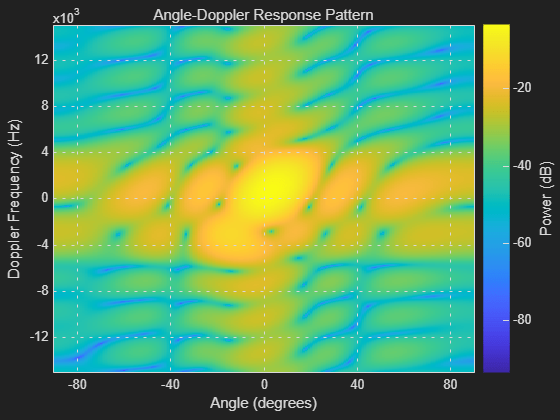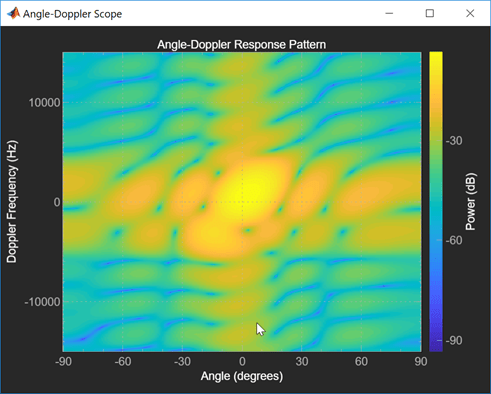# phased.AngleDopplerScope

Angle-Doppler scope

## Description

The `phased.AngleDopplerScope` System object™ creates a scope for displaying an angle-Doppler response map. The map is a 2-D representation of response intensity as a function of angle and Doppler shift. You can input two types of data - in-phase and quadrature (I/Q) data and response data.

• I/Q data – The data consists of I/Q samples at the same range from multiple sensors over all pulses or sweeps. The scope computes and displays the response map. To use I/Q data, set the `IQDataInput` property to `true`. In this mode, you can set the properties listed in Properties Applicable to I/Q Data.

• Response data – The data consists of the angle-Doppler response itself. The scope only displays the angle-Doppler response map. You can obtain angle-Doppler response data from the `phased.AngleDopplerResponse` object. To display response data, set the `IQDataInput` property to `false`. In this mode, you can set the properties listed in Properties Applicable to Response Data.To display an angle-Doppler response map using a scope,

1. Create the `phased.AngleDopplerScope` object and set its properties.

2. Call the object with arguments, as if it were a function.

## Creation

### Syntax

``scope = phased.AngleDopplerScope``
``scope = phased.phased.AngleDopplerScope(Name,Value)``

### Description

````scope = phased.AngleDopplerScope` creates an angle-Doppler `scope` System object for displaying the angle-Doppler response map.```

example

````scope = phased.phased.AngleDopplerScope(Name,Value)`creates an angle-Doppler `scope` with each specified property set to the specified value. You can specify additional name-value pair arguments in any order as (`Name1`,`Value1`,...,`NameN`,`ValueN`). Enclose property names in quotes. For example,scope = phased.AngleDopplerScope('IQInputData',true, ... 'NumAngleSamples',128,'NumDopplerSamples',64)creates a scope object that computes and displays the angle-Doppler response at 128 angle values and 64 Doppler values from I/Q data input.```

## Properties

expand all

Unless otherwise indicated, properties are nontunable, which means you cannot change their values after calling the object. Objects lock when you call them, and the `release` function unlocks them.

If a property is tunable, you can change its value at any time.

Display caption, specified as a character vector. The caption appears in the title bar of the window.

Example: `'Aircraft Angle-Doppler Response'`

Tunable: Yes

Data Types: `char`

Location and size of the intensity scope window, specified as a 1-by-4 vector having the form `[left bottom width height]`.

• `left` and `bottom` specify the location of the bottom-left corner of the window.

• `width` and `height` specify the width and height of the window.

Units are in pixels.

The default value of this property depends on the resolution of your display. By default, the window is positioned in the center of the screen, with a width and height of 800 and 450 pixels, respectively.

Example: `[100 100 500 400]`

Tunable: Yes

Data Types: `double`

Type of input data, specified as `true` or `false`. When `true`, the object assumes that the input consists of I/Q sample data and further processing is required in the range, angle, or Doppler domains. When `false`, the object assumes that the data is response data that has already been processed.

Data Types: `logical`

Response units, specified as `'db'`, `'magnitude'`, or `'power'`.

Data Types: `char`

Angle-axis label, specified as a character vector.

Example: `'Angle Span (degrees)'`

Tunable: Yes

#### Dependencies

To enable this property, set the `IQDataInput` to `false`.

Data Types: `char`

Doppler-axis label, specified as a character vector.

Example: `'Doppler Frequency (kHz)'`

Tunable: Yes

#### Dependencies

To enable this property, set the `IQDataInput` to `false`.

Data Types: `char`

Sensor array, specified as a Phased Array System Toolbox array System object. See `phased.ULA` for the default values of a uniform linear array.

Example: `phased.URA`

#### Dependencies

To enable this property, set the `IQDataInput` to `true`.

Signal propagation speed, specified as a positive scalar. The default value of this property is the speed of light. See `physconst`. Units are in meters/second.

Example: `3e8`

#### Dependencies

To enable this property, set the `IQDataInput` property to `true`.

Data Types: `double`

Operating frequency, specified as a positive scalar. Units are in Hz.

#### Dependencies

To enable this property, set the `IQDataInput` property to `true`.

Data Types: `double`

Pulse repetition frequency of input signal, specified as a positive scalar. Units are in Hz.

Example: `1.4e3`

#### Dependencies

To enable this property, set the `IQDataInput` property to `true`.

Data Types: `double`

Elevation angle at which to calculate the response, specified as a scalar. The elevation angle must lie in the interval from –90° to 90°, inclusive. Units are in degrees.

Example: `45.0`

#### Dependencies

To enable this property, set the `IQDataInput` property to `true`.

Data Types: `double`

Number of bins in the angle span at which to calculate the response, specified as a positive integer greater than two.

Example: `256`

#### Dependencies

To enable this property, set the `IQDataInput` property to `true`.

Data Types: `double`

Number of bins in the Doppler domain used to calculate angle-Doppler response, specified as a positive integer greater than two.

Example: `512`

#### Dependencies

To enable this property, set the `IQDataInput` property to `true`.

Data Types: `double`

Set this property to `true` to plot the angle-Doppler response at the normalized Doppler frequency. Set this property to `false` to plot the angle-Doppler response without normalizing the Doppler frequency.

#### Dependencies

To enable this property, set the `IQDataInput` property to `true`.

Data Types: `logical`

Doppler frequency units, specified as `'Hz'`, `'kHz'`, or `'MHz'`.

Example: `'MHz'`

#### Dependencies

To enable this property, set the `IQDataInput` property to `true` and the `NormalizedDoppler` property to `false`.

Data Types: `char`

## Usage

### Syntax

``scope(X,Ang,Dop)``
``scope(X)``

### Description

````scope(X,Ang,Dop)` displays an angle-Doppler response map for the response data, `scope`, for direction azimuth angles, `Ang`, and Doppler shifts, `Dop`. This syntax applies when you set the `IQDataInput` to `false`.```
````scope(X)` computes and displays the angle-Doppler response map of the I/Q data `X`. This syntax applies when you set the `IQDataInput` property to `true`.```

### Input Arguments

expand all

Input data, specified as a real-valued P-by-Q or complex-valued P-by-Q matrix. The processing of the data depends on the value of the `IQDataInput` property.

• When `IQDataInput` is `true`, `x` consists of I/Q samples at fixed range of pulses or sweeps from multiple elements or subarrays. P is the number of array elements. If `SensorArray` contains subarrays, P is the number of subarrays. Q is the number of pulses. The scope computes and displays the angle-Doppler response.

When `x` is a column vector, L must be equal to an integer multiple of P.

• When `IQDataInput` is `false`, `x` consists of real-valued angle-Doppler response data such as the data produced by `phased.AngleDopplerResponse`. P is the number of Doppler samples and Q is the number of angle samples. Each row represents a Doppler value corresponding to an element of `Dop`. Each column represents an angle value corresponding to an element of the `Ang` vector. The scope serves only as a display of the angle-Doppler response.

Azimuth angle grid values of response map, specified as a real-valued Q-by-1 column vector. `Ang` contains the angle values corresponding to the columns of `X`.

#### Dependencies

To enable this argument, set the `IQInputData` property to `false`.

Data Types: `double`

Doppler grid values of response map, specified as a real-valued P-by-1 column vector. `Dop` contains the Doppler values corresponding to the rows of `X`.

#### Dependencies

To enable this argument, set the `IQInputData` property to `false`.

Data Types: `double`

## Object Functions

To use an object function, specify the System object as the first input argument. For example, to release system resources of a System object named `obj`, use this syntax:

`release(obj)`

expand all

 `show` Turn on visibility of scopes `hide` Turn off visibility of scope `isVisible` Visibility of scopes
 `step` Run System object algorithm `release` Release resources and allow changes to System object property values and input characteristics `reset` Reset internal states of System object

## Examples

collapse all

Calculate and visualize the angle-Doppler response at a single range cell of a collected data cube.

Load the I/Q data and analyze the 43th range cell.

```load STAPExampleData; x = shiftdim(STAPEx_ReceivePulse(43,:,:));```

Create a scope object that processes I/Q data.

```scope = phased.AngleDopplerScope( ... 'IQDataInput', true, ... 'Name','Angle-Doppler Scope', ... 'Position',[560 375 560 420], ... 'NormalizeDoppler',false, ... 'ResponseUnits','db', ... 'SensorArray',STAPEx_HArray, ... 'OperatingFrequency',STAPEx_OperatingFrequency, ... 'PropagationSpeed',STAPEx_PropagationSpeed, ... 'PRF',STAPEx_PRF,'NumDopplerSamples',512);```

Compute and visualize the angle-Doppler response.

`scope(x)`